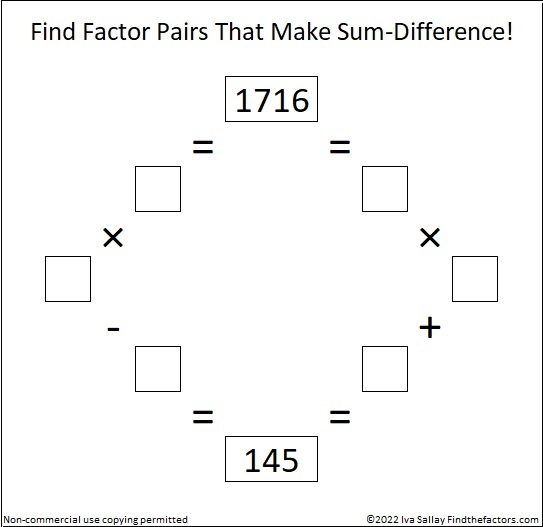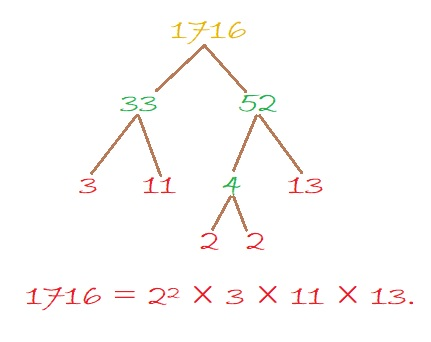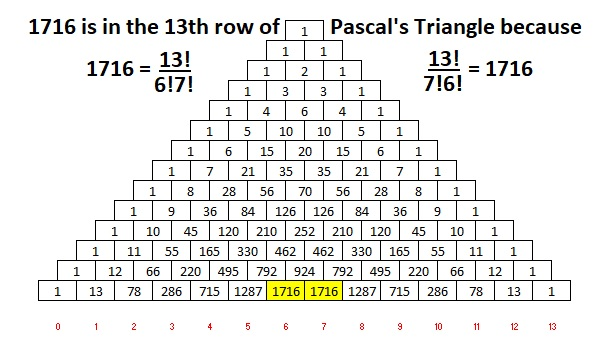# Two Factor Pairs of 1716 Make Sum-Difference!

Contents

### Today’s Puzzle:

How many factor pairs does 1716 have? Twelve. The factors in one of those factor pairs add up to 145 and the factors in a different one subtract to 145. If you can find those two factor pairs, then you can solve this puzzle!Try to solve it all by yourself, but if you need some help, you can scroll down to learn more about the factors of 1716.

### A Factor Tree for 1716:

3 is a factor of 1716 because 1 + 7 + 1 + 6 = 15, a multiple of 3.
11 is a factor of 1716 because 1 – 7 + 1 – 6 = -11.

3 ·11 is 33. Here is one of MANY possible factor trees for 1716. This one uses the fact that 33 · 52 = 1716:### Factors of 1716:

• 1716 is a composite number.
• Prime factorization: 1716 = 2 × 2 × 3 × 11 × 13, which can be written 1716 = 2² × 3 × 11 × 13.
• 1716 has at least one exponent greater than 1 in its prime factorization so √1716 can be simplified. Taking the factor pair from the factor pair table below with the largest square number factor, we get √1716 = (√4)(√429) = 2√429.
• The exponents in the prime factorization are 2, 1, 1, and 1. Adding one to each exponent and multiplying we get (2 + 1)(1 + 1)(1 + 1)(1 + 1) = 3 × 2 × 2 × 2 = 24. Therefore 1716 has exactly 24 factors.
• The factors of 1716 are outlined with their factor pair partners in the graphic below.Here’s a chart of those same factor pairs but in reverse order with their sums and differences included.### More About the Number 1716:

1716 is the hypotenuse of a Pythagorean triple:
660-1584-1716, which is (5-12-13) times 132.

OEIS.org reminds us that 1716 is in the 6th and 7th columns of the 13th row of Pascal’s Triangle:This site uses Akismet to reduce spam. Learn how your comment data is processed.